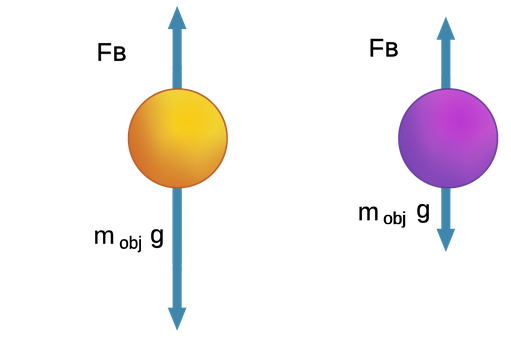If we hold an object completely below the surface of the liquid (e.g. underwater), we have seen that the buoyant force on it depends on its volume Vobject and the density of the fluid: FB = ρfluid · Vobject · g

The question is whether the object will sink or rise up to the surface when released. That depends on whether the object’s weight, mobject · g is greater than or less than the buoyant force.

If this weight is greater than the buoyant force, it will sink below the surface.

The condition for an object to sink is: m · g > FB, i.e., mobject · g > ρfluid · Vobject · g .

Rearranging and canceling the “g”, we find the condition mobject /Vobject > ρfluid. On the left side, the mass of the object divided by its volume is just its average density, ρobject.

So we can write for an object that sinks, ρobject> ρfluid, i.e., if the object’s density is greater than the liquid’s, it will sink to the bottom.

On the other hand, if the weight of our completely immersed object is less than the buoyant force on it, the object will experience a net force upwards, causing it to rise upwards to the surface. Once at the surface, the object will float with just a fraction of its volume submerged, such that the buoyant force and its weight are in equilibrium.

The condition for an object that floats: m · g < FB, i.e. mobject · g < ρfluid · Vobject · g. As before, rearranging and canceling the “g”gives us the condition for flotation:

mobject /Vobject = ρobject > ρfluid

This means that if the object’s average density is less than the liquid’s it will rise up and float on the surface.Figure 1:Two balls underwater, with equal volumes but different weights. On the left, the ball weighs more than the buoyant force, so it sinks. On the right, the ball weighs less than the buoyant force, so it rises to the surface.